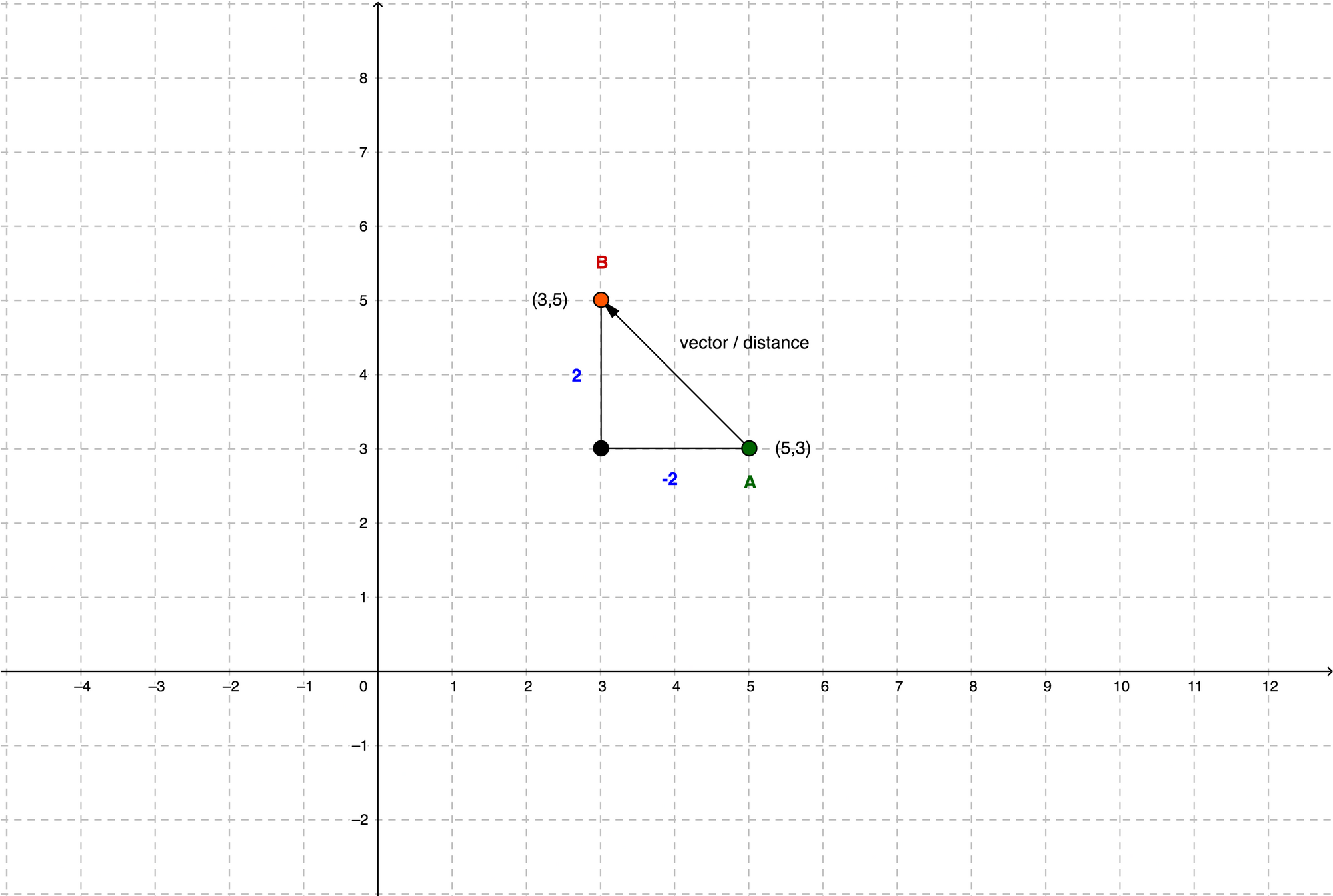# Basic Math Theory for Game Development | VECTORS

In this story, we’ll look at one of the essential concepts in game development, it’s the vector. This is why it’s useful in game development. It’s often used when we want to move a character in a scene.

Let’s say we want to move it 4 units to the right & 4 units up. We create a vector that is represented by the arrow that points in the direction where our character needs to go. Mathematically the vector is (4,4), 4 on X-axis & 4 on Y-axis.

We can then add this vector to the current position of the character which is (3,2). So the next position that our character should be after we add this vector is (7,6).

## What is a vector

A vector is defined by direction and length, that’s why we used an arrow to represent a vector. It can be directed anywhere in the space right, left, up, and down. The distance of a vector shows how far the character goes in space.

A vector also doesn't have any inherent position which means it can be placed anywhere we want because it’s just a direction with length.

## How do I use vector in game dev?

Here is another example where vectors come in handy.A vector (2,2) or distance between 2 players A & B.

When we have two players in the scene and we want to get the distance or length between these two players. Both players have their own positions that are (5,3) for player A and (3,5) for player B from the center respectively. Remember vector is direction and length at the same time. So length is equal to distance. How can we calculate the distance between these 2 players?

## Calculate the vector

To do this we subtract the position of player A from the position of player B or vice versa because we just want to get the distance between players. It can be done just like this(3,5)-(5,3). We subtract the x component 3–5=-2 then we subtract the y component 5–3=2 and the result is a vector of (-2,2), But how do we calculate the length of this vector?

That is really easy. It’s in fact the Pythagoras Theorem. The hypotenuse is the length of a vector or referred to as the magnitude.

So if we want to calculate the distance between our players, we simply plug in the vector (-2,2) into the formula Square root((-2)² + 2²) = 2.82842713. The distance between players A & B is 2.83 units. This is the same in 3d as well, it just has the third component added, which is the z-axis (x,y,z). Just add another one to the formula, then it would be like this: Square root(x² + y² + z²).

## Why do I need to know about vectors?

Yeah, our game engine has an easy way to calculate this. Unity has an easy function of getting the distance between two points in space like Vector3.Distance(A, B); then why do we need to know about this?

Well, this knowledge is super useful when it comes to optimizing your code. Say we want to compare the distance of two players from the finish line. We want to know which is ahead. So use this formula Square root(x² + y²) twice in order to get two vectors.